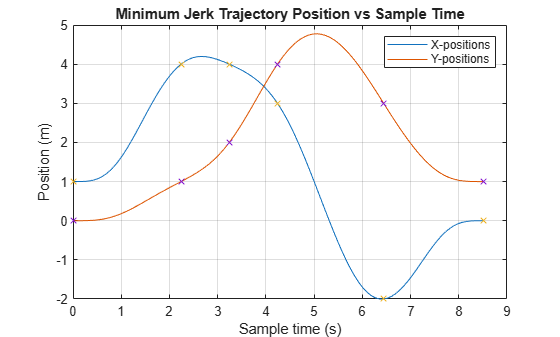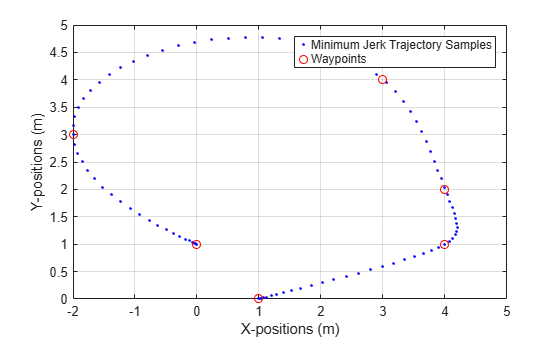# minjerkpolytraj

Generate minimum jerk trajectory through waypoints

## Syntax

``[q,qd,qdd,qddd,pp,tPoints,tSamples] = minjerkpolytraj(waypoints,timePoints,numSamples)``
``[q,qd,qdd,qddd,pp,tPoints,tSamples] = minjerkpolytraj(___,Name=Value)``
``[q,qd,qdd,qddd,pp,tPoints,tSamples] = minjerkpolytraj(___,TimeAllocation=true)``

## Description

example

````[q,qd,qdd,qddd,pp,tPoints,tSamples] = minjerkpolytraj(waypoints,timePoints,numSamples)` generates a minimum jerk polynomial trajectory that achieves a given set of input waypoints with their corresponding time points. The function returns positions, velocities, accelerations, and jerks at the given number of samples `numSamples`. The function also returns the piecewise polynomial `pp` form of the polynomial trajectory with respect to time, as well as the time points `tPoints` and the sample times `tSamples`.```
````[q,qd,qdd,qddd,pp,tPoints,tSamples] = minjerkpolytraj(___,Name=Value)` specifies options using one or more name-value pair arguments in addition to the input arguments from the previous syntax. For example, ```minjerkpolytraj(waypoints,timePoints,numSamples,VelocityBoundaryCondition=[1 0 -1 -1; 1 1 1 -1])``` generates a two-dimensional minimum jerk trajectory and specifies the velocity boundary conditions in each dimension for each waypoint.```
````[q,qd,qdd,qddd,pp,tPoints,tSamples] = minjerkpolytraj(___,TimeAllocation=true)` optimizes a combination of jerk and total segment time cost. In this case, the function treats `timePoints` as an initial guess for the time of arrival at the waypoints.```

## Examples

collapse all

Use the `minjerkpolytraj` function with a given set of 2-D xy waypoints. Time points for the waypoints are also given.

```wpts = [1 4 4 3 -2 0; 0 1 2 4 3 1]; tpts = 0:5;```

Specify the number of samples in the output trajectory.

`numsamples = 100;`

Compute minimum jerk trajectories. The function outputs the trajectory positions (`q`), velocity (`qd`), acceleration (`qdd`), and jerks (`qddd`) at the given number of samples.

`[q,qd,qdd,qddd,pp,timepoints,tsamples] = minjerkpolytraj(wpts,tpts,numsamples);`

Plot the trajectories for the x- and y-positions. Compare the trajectory with each waypoint.

```plot(tsamples,q) hold on plot(timepoints,wpts,'x') xlabel('t') ylabel('Positions') legend('X-positions','Y-positions') hold off```You can also verify the actual positions in the 2-D plane. Plot the separate rows of the `q` vector and the waypoints as x- and y- positions.

```figure plot(q(1,:),q(2,:),'.b',wpts(1,:),wpts(2,:),'or') xlabel('X') ylabel('Y')```## Input Arguments

collapse all

Waypoints for the trajectory, specified as an n-by-p matrix. n is the dimension of the trajectory, and p is the number of waypoints.

Example: `[2 5 8 4; 3 4 10 12]`

Data Types: `single` | `double`

Time points for the waypoints of the trajectory, specified as a p-element row vector. p is the number of waypoints.

Example: `[1 2 3 5]`

Data Types: `single` | `double`

Number of samples in the output trajectory, specified as a positive integer.

Example: `50`

Data Types: `single` | `double`

### Name-Value Arguments

Specify optional pairs of arguments as `Name1=Value1,...,NameN=ValueN`, where `Name` is the argument name and `Value` is the corresponding value. Name-value arguments must appear after other arguments, but the order of the pairs does not matter.

Example: ```minjerkpolytraj(waypoints,timePoints,numSamples,VelocityBoundaryCondition=[1 0 -1 -1; 1 1 1 -1])``` generates a two-dimensional minimum jerk trajectory and specifies the velocity boundary conditions in each dimension for each waypoint.

Velocity boundary conditions for each waypoint, specified as an n-by-p matrix. Each row sets the velocity boundary for the corresponding dimension of the trajectory at each of p waypoints. By default, the function uses a value of `0` at the boundary waypoints and `NaN` at the intermediate waypoints.

Example: ```VelocityBoundaryCondition=[1 0 -1 -1; 1 1 1 -1]```

Data Types: `single` | `double`

Acceleration boundary conditions for each waypoint, specified as an n-by-p matrix. Each row sets the acceleration boundary for the corresponding dimension of the trajectory at each of p waypoints. By default, the function uses a value of `0` at the boundary waypoints and `NaN` at the intermediate waypoints.

Example: ```AccelerationBoundaryCondition=[1 0 -1 -1; 1 1 1 -1]```

Data Types: `single` | `double`

Jerk boundary conditions for each waypoint, specified as an n-by-p matrix. Each row sets the jerk boundary for the corresponding dimension of the trajectory at each of p waypoints. By default, the function uses a value of `0` at the boundary waypoints and `NaN` at the intermediate waypoints.

Example: ```JerkBoundaryCondition=[1 0 -1 -1; 1 1 1 -1]```

Data Types: `single` | `double`

Time allocation flag, specified as a logical `0` (`false`) or `1` (`true`). Enable this flag to optimize a combination of jerk and total segment time cost.

Note

If singularity occurs when the time allocation flag is enabled, reduce the `MaxSegmentTime` to `MinSegmentTime` ratio.

Example: `TimeAllocation=true`

Data Types: `logical`

Weight for time allocation, specified as a positive scalar.

Example: `TimeWeight=120`

Data Types: `single` | `double`

Minimum time segment length, specified as a positive scalar or (p`–``1`)-element row vector.

Example: `MinSegmentTime=0.2`

Data Types: `single` | `double`

Maximum time segment length, specified as a positive scalar or (p`–``1`)-element row vector

Example: `MaxSegmentTime=10`

Data Types: `single` | `double`

## Output Arguments

collapse all

Positions of the trajectory at the given time samples in `tSamples`, returned as an n-by-m matrix. n is the dimension of the trajectory, and m is equal to `numSamples`.

Velocities of the trajectory at the given time samples in `tSamples`, returned as an n-by-m matrix. n is the dimension of the trajectory, and m is equal to `numSamples`.

Accelerations of the trajectory at the given time samples in `tSamples`, returned as an n-by-m matrix. n is the dimension of the trajectory, and m is equal to `numSamples`.

Jerks of the trajectory at the given time samples in `tSamples`, returned as an n-by-m matrix. n is the dimension of the trajectory, and m is equal to `numSamples`.

Piecewise-polynomial, returned as a structure that defines the polynomial for each section of the piecewise trajectory. You can build your own piecewise polynomials using `mkpp`, or evaluate the polynomial at specified times using `ppval`. The structure contains the fields:

• `form`: `'pp'`.

• `breaks`: p-element vector of times when the piecewise trajectory changes forms. p is the number of waypoints.

• `coefs`: n(p`–``1`)-by-`order` matrix for the coefficients for the polynomials. n(p`–``1`) is the dimension of the trajectory times the number of `pieces`. Each set of n rows defines the coefficients for the polynomial that described each variable trajectory.

• `pieces`: p`–``1`. The number of breaks minus 1.

• `order`: Degree of the polynomial `+` `1`. The order of polynomial is 8.

• `dim`: n. The dimension of the control point positions.

Time points for the waypoints of the trajectory, returned as a p-element row vector. p is the number of waypoints.

Time samples for the trajectory, returned as an m-element row vector. Each element of the output position `q`, velocity `qd`, acceleration `qdd`, and jerk `qddd` has been sampled at the corresponding time in this vector.

 Bry, Adam, Charles Richter, Abraham Bachrach, and Nicholas Roy. “Aggressive Flight of Fixed-Wing and Quadrotor Aircraft in Dense Indoor Environments.” The International Journal of Robotics Research, 34, no. 7 (June 2015): 969–1002.

 Richter, Charles, Adam Bry, and Nicholas Roy. “Polynomial Trajectory Planning for Aggressive Quadrotor Flight in Dense Indoor Environments." Paper presented at the International Symposium of Robotics Research (ISRR 2013), 2013.